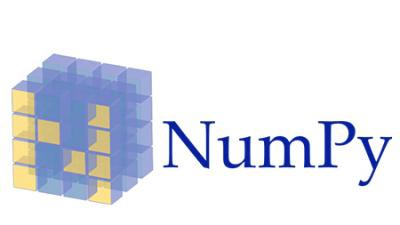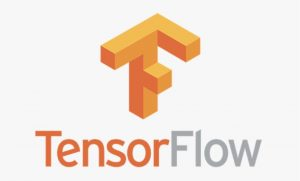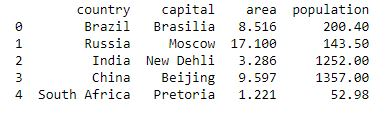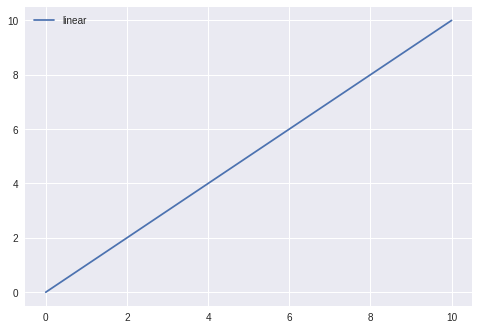Related Articles
Best Python libraries for Machine Learning
• Difficulty Level : Easy
• Last Updated : 23 Aug, 2019

Machine Learning, as the name suggests, is the science of programming a computer by which they are able to learn from different kinds of data. A more general definition given by Arthur Samuel is – “Machine Learning is the field of study that gives computers the ability to learn without being explicitly programmed.” They are typically used to solve various types of life problems.
In the older days, people used to perform Machine Learning tasks by manually coding all the algorithms and mathematical and statistical formula. This made the process time consuming, tedious and inefficient. But in the modern days, it is become very much easy and efficient compared to the olden days by various python libraries, frameworks, and modules. Today, Python is one of the most popular programming languages for this task and it has replaced many languages in the industry, one of the reason is its vast collection of libraries. Python libraries that used in Machine Learning are:

• Numpy
• Scipy
• Scikit-learn
• Theano
• TensorFlow
• Keras
• PyTorch
• Pandas
• Matplotlib

#### NumpyNumPy is a very popular python library for large multi-dimensional array and matrix processing, with the help of a large collection of high-level mathematical functions. It is very useful for fundamental scientific computations in Machine Learning. It is particularly useful for linear algebra, Fourier transform, and random number capabilities. High-end libraries like TensorFlow uses NumPy internally for manipulation of Tensors.

 `# Python program using NumPy ``# for some basic mathematical ``# operations`` ` `import` `numpy as np`` ` `# Creating two arrays of rank 2``x ``=` `np.array([[``1``, ``2``], [``3``, ``4``]])``y ``=` `np.array([[``5``, ``6``], [``7``, ``8``]])`` ` `# Creating two arrays of rank 1``v ``=` `np.array([``9``, ``10``])``w ``=` `np.array([``11``, ``12``])`` ` `# Inner product of vectors``print``(np.dot(v, w), ``"\n"``)`` ` `# Matrix and Vector product``print``(np.dot(x, v), ``"\n"``)`` ` `# Matrix and matrix product``print``(np.dot(x, y))`

Output:

```219

[29 67]

[[19 22]
[43 50]]
```

For more details refer to Numpy.

#### SciPySciPy is a very popular library among Machine Learning enthusiasts as it contains different modules for optimization, linear algebra, integration and statistics. There is a difference between the SciPy library and the SciPy stack. The SciPy is one of the core packages that make up the SciPy stack. SciPy is also very useful for image manipulation.

 `# Python script using Scipy ``# for image manipulation`` ` `from` `scipy.misc ``import` `imread, imsave, imresize`` ` `# Read a JPEG image into a numpy array``img ``=` `imread(``'D:/Programs / cat.jpg'``) ``# path of the image``print``(img.dtype, img.shape)`` ` `# Tinting the image``img_tint ``=` `img ``*` `[``1``, ``0.45``, ``0.3``]`` ` `# Saving the tinted image``imsave(``'D:/Programs / cat_tinted.jpg'``, img_tint)`` ` `# Resizing the tinted image to be 300 x 300 pixels``img_tint_resize ``=` `imresize(img_tint, (``300``, ``300``))`` ` `# Saving the resized tinted image``imsave(``'D:/Programs / cat_tinted_resized.jpg'``, img_tint_resize)`

Original image:Tinted image:Resized tinted image:For more details refer to documentation.

#### Scikit-learnSkikit-learn is one of the most popular ML libraries for classical ML algorithms. It is built on top of two basic Python libraries, viz., NumPy and SciPy. Scikit-learn supports most of the supervised and unsupervised learning algorithms. Scikit-learn can also be used for data-mining and data-analysis, which makes it a great tool who is starting out with ML.

 `# Python script using Scikit-learn ``# for Decision Tree Clasifier`` ` `# Sample Decision Tree Classifier``from` `sklearn ``import` `datasets``from` `sklearn ``import` `metrics``from` `sklearn.tree ``import` `DecisionTreeClassifier`` ` `# load the iris datasets``dataset ``=` `datasets.load_iris()`` ` `# fit a CART model to the data``model ``=` `DecisionTreeClassifier()``model.fit(dataset.data, dataset.target)``print``(model)`` ` `# make predictions``expected ``=` `dataset.target``predicted ``=` `model.predict(dataset.data)`` ` `# summarize the fit of the model``print``(metrics.classification_report(expected, predicted))``print``(metrics.confusion_matrix(expected, predicted))`

Output:

```DecisionTreeClassifier(class_weight=None, criterion='gini', max_depth=None,
max_features=None, max_leaf_nodes=None,
min_impurity_decrease=0.0, min_impurity_split=None,
min_samples_leaf=1, min_samples_split=2,
min_weight_fraction_leaf=0.0, presort=False, random_state=None,
splitter='best')
precision    recall  f1-score   support

0       1.00      1.00      1.00        50
1       1.00      1.00      1.00        50
2       1.00      1.00      1.00        50

micro avg       1.00      1.00      1.00       150
macro avg       1.00      1.00      1.00       150
weighted avg       1.00      1.00      1.00       150

[[50  0  0]
[ 0 50  0]
[ 0  0 50]]
```

For more details refer to documentation.

#### TheanoWe all know that Machine Learning is basically mathematics and statistics. Theano is a popular python library that is used to define, evaluate and optimize mathematical expressions involving multi-dimensional arrays in an efficient manner. It is achieved by optimizing the utilization of CPU and GPU. It is extensively used for unit-testing and self-verification to detect and diagnose different types of errors. Theano is a very powerful library that has been used in large-scale computationally intensive scientific projects for a long time but is simple and approachable enough to be used by individuals for their own projects.

 `# Python program using Theano``# for computing a Logistic ``# Function`` ` `import` `theano``import` `theano.tensor as T``x ``=` `T.dmatrix(``'x'``)``s ``=` `1` `/` `(``1` `+` `T.exp(``-``x))``logistic ``=` `theano.function([x], s)``logistic([[``0``, ``1``], [``-``1``, ``-``2``]])`

Output:

```array([[0.5, 0.73105858],
[0.26894142, 0.11920292]])
```

For more details refer to documentation.

#### TensorFlowTensorFlow is a very popular open-source library for high performance numerical computation developed by the Google Brain team in Google. As the name suggests, Tensorflow is a framework that involves defining and running computations involving tensors. It can train and run deep neural networks that can be used to develop several AI applications. TensorFlow is widely used in the field of deep learning research and application.

 `#  Python program using TensorFlow``#  for multiplying two arrays`` ` `# import `tensorflow` ``import` `tensorflow as tf`` ` `# Initialize two constants``x1 ``=` `tf.constant([``1``, ``2``, ``3``, ``4``])``x2 ``=` `tf.constant([``5``, ``6``, ``7``, ``8``])`` ` `# Multiply``result ``=` `tf.multiply(x1, x2)`` ` `# Initialize the Session``sess ``=` `tf.Session()`` ` `# Print the result``print``(sess.run(result))`` ` `# Close the session``sess.close()`

Output:

```[ 5 12 21 32]
```

For more details refer to documentation.

#### KerasKeras is a very popular Machine Learning library for Python. It is a high-level neural networks API capable of running on top of TensorFlow, CNTK, or Theano. It can run seamlessly on both CPU and GPU. Keras makes it really for ML beginners to build and design a Neural Network. One of the best thing about Keras is that it allows for easy and fast prototyping.

For more details refer to documentation.

#### PyTorchPyTorch is a popular open-source Machine Learning library for Python based on Torch, which is an open-source Machine Learning library which is implemented in C with a wrapper in Lua. It has an extensive choice of tools and libraries that supports on Computer Vision, Natural Language Processing(NLP) and many more ML programs. It allows developers to perform computations on Tensors with GPU acceleration and also helps in creating computational graphs.

 `# Python program using PyTorch ``# for defining tensors fit a ``# two-layer network to random ``# data and calculating the loss `` ` `import` `torch`` ` ` ` `dtype ``=` `torch.``float``device ``=` `torch.device(``"cpu"``)``# device = torch.device("cuda:0") Uncomment this to run on GPU`` ` `# N is batch size; D_in is input dimension;``# H is hidden dimension; D_out is output dimension.``N, D_in, H, D_out ``=` `64``, ``1000``, ``100``, ``10`` ` `# Create random input and output data``x ``=` `torch.randn(N, D_in, device ``=` `device, dtype ``=` `dtype)``y ``=` `torch.randn(N, D_out, device ``=` `device, dtype ``=` `dtype)`` ` `# Randomly initialize weights``w1 ``=` `torch.randn(D_in, H, device ``=` `device, dtype ``=` `dtype)``w2 ``=` `torch.randn(H, D_out, device ``=` `device, dtype ``=` `dtype)`` ` `learning_rate ``=` `1e``-``6``for` `t ``in` `range``(``500``):``    ``# Forward pass: compute predicted y``    ``h ``=` `x.mm(w1)``    ``h_relu ``=` `h.clamp(``min` `=` `0``)``    ``y_pred ``=` `h_relu.mm(w2)`` ` `    ``# Compute and print loss``    ``loss ``=` `(y_pred ``-` `y).``pow``(``2``).``sum``().item()``    ``print``(t, loss)`` ` `    ``# Backprop to compute gradients of w1 and w2 with respect to loss``    ``grad_y_pred ``=` `2.0` `*` `(y_pred ``-` `y)``    ``grad_w2 ``=` `h_relu.t().mm(grad_y_pred)``    ``grad_h_relu ``=` `grad_y_pred.mm(w2.t())``    ``grad_h ``=` `grad_h_relu.clone()``    ``grad_h[h < ``0``] ``=` `0``    ``grad_w1 ``=` `x.t().mm(grad_h)`` ` `    ``# Update weights using gradient descent``    ``w1 ``-``=` `learning_rate ``*` `grad_w1``    ``w2 ``-``=` `learning_rate ``*` `grad_w2`

Output:

```0 47168344.0
1 46385584.0
2 43153576.0
...
...
...
497 3.987660602433607e-05
498 3.945609932998195e-05
499 3.897604619851336e-05
```

For more details refer to documentation.

#### PandasPandas is a popular Python library for data analysis. It is not directly related to Machine Learning. As we know that the dataset must be prepared before training. In this case, Pandas comes handy as it was developed specifically for data extraction and preparation. It provides high-level data structures and wide variety tools for data analysis. It provides many inbuilt methods for groping, combining and filtering data.

 `# Python program using Pandas for ``# arranging a given set of data ``# into a  table`` ` `# importing pandas as pd``import` `pandas as pd`` ` `data ``=` `{``"country"``: [``"Brazil"``, ``"Russia"``, ``"India"``, ``"China"``, ``"South Africa"``],``       ``"capital"``: [``"Brasilia"``, ``"Moscow"``, ``"New Dehli"``, ``"Beijing"``, ``"Pretoria"``],``       ``"area"``: [``8.516``, ``17.10``, ``3.286``, ``9.597``, ``1.221``],``       ``"population"``: [``200.4``, ``143.5``, ``1252``, ``1357``, ``52.98``] }`` ` `data_table ``=` `pd.DataFrame(data)``print``(data_table)`

Output:For more details refer to Pandas.

#### MatplotlibMatpoltlib is a very popular Python library for data visualization. Like Pandas, it is not directly related to Machine Learning. It particularly comes in handy when a programmer wants to visualize the patterns in the data. It is a 2D plotting library used for creating 2D graphs and plots. A module named pyplot makes it easy for programmers for plotting as it provides features to control line styles, font properties, formatting axes, etc. It provides various kinds of graphs and plots for data visualization, viz., histogram, error charts, bar chats, etc,

 `#  Python program using Matplotib ``# for forming a linear plot`` ` `# importing the necessary packages and modules``import` `matplotlib.pyplot as plt``import` `numpy as np`` ` `# Prepare the data``x ``=` `np.linspace(``0``, ``10``, ``100``)`` ` `# Plot the data``plt.plot(x, x, label ``=``'linear'``)`` ` `# Add a legend``plt.legend()`` ` `# Show the plot``plt.show()`

Output:For more details refer to documentation.My Personal Notes arrow_drop_up